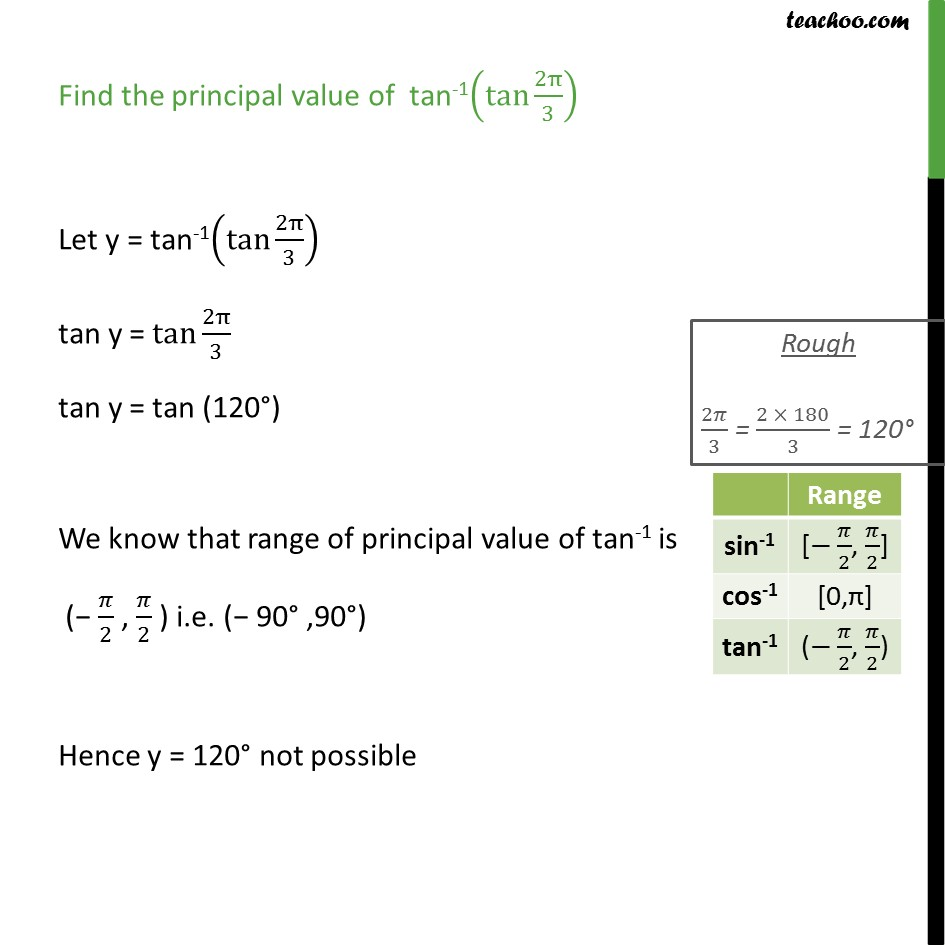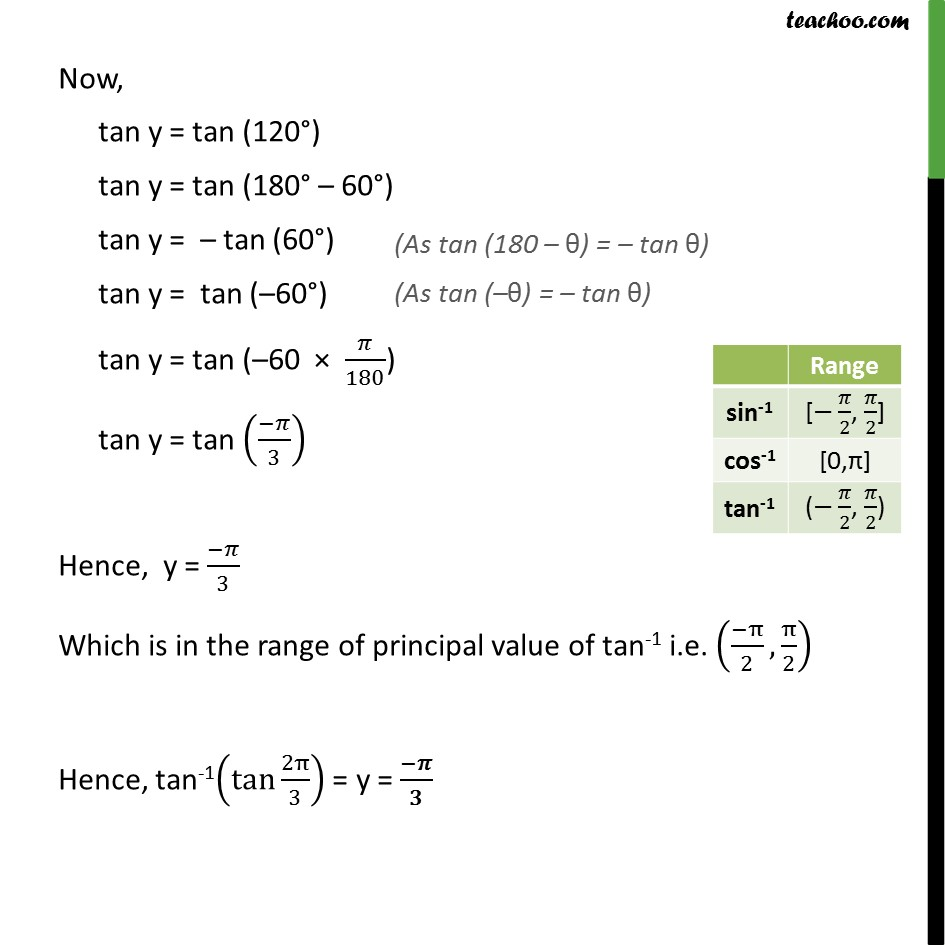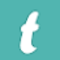## Find principal value of tan-1 (tan 2π÷3)The text version of the answer is -

Find the principal value of  tan-1(tan⁡〖2π/3〗 )

Let y = tan-1(tan⁡〖2π/3〗 )

tan y =〖 tan〗⁡〖2π/3〗

tan y = tan (120°)

We know that range of principal value of tan-1 is

(− π/2 , π/2 ) i.e. (− 90° ,90°)

Hence y = 120° not possible

Now,

tan y = tan (120°)

tan y = tan (180° – 60°)

tan y =  – tan (60°)

tan y =  tan (–60°)

tan y = tan (–60  ×  π/180)

tan y = tan ((-π)/3)

Hence,  y = (-π)/3

Which is in the range of principal value of tan-1 i.e. ((-π)/2, π/2)

Hence, tan-1(tan⁡〖2π/3〗 ) = y = ( - π)/ 3

Notes -

(As tan (180 θ ) = – tan θ )

(As tan (– θ ) = – tan θ )

 Range sin -1 [-π/2, π/2] cos -1 [0,π] tan -1 (-π/2, π/2)